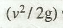# Hydraulic Gradient and Total Energy Lines

The line representing the sum of pressure head (p/w) and potential head or datum head (z) with respect to some reference line is hydraulic gradient line (H.G.L).

The line representing the sum of pressure head (p/w), datum head (z) and velocity head with respect to some reference line is known as total energy line (T.G.L). Velocity head is given by: